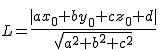## Distance from a point to a plane calculator

 Enter the point , , Plane Equation x+ y+ z+ =0 Shortest distance between point and plane

The Distance from a point to a plane calculator to find the shortest distance between a point and the plane

### FormulaWhere point (x0,y0,z0), Plane (ax+by+cz+d=0)

For example, Give the point (2,-3,1) and the plane 3x+y-2z=15

Distance = |ax+by+cz-d| / sqrt(a^2 + b^2 + c^2) = |3(2) + (-3) + (-2) - 15| / sqrt(a^2 + b^2 + c^2) = |-14| / sqrt14 = 3.7416573867739413

Thinkcalculator.com provides you helpful and handy calculator resources.